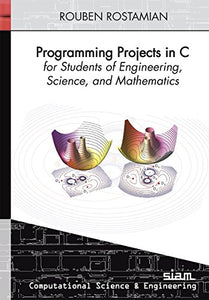# Programming Projects In C For Students Of Engineering, Science, And Mathematics

• Publish Date: 2014-09-03
• Binding: Paperback
• Author: Rouben Rostamian
Regular price \$94.90 Sale price \$91.67

Attention: For textbook, access codes and supplements are not guaranteed with used items.

Like a pianist who practices from a book of tudes, readers of Programming Projects in C for Students of Engineering, Science, and Mathematics will learn by doing.
Written as a tutorial on how to think about, organize, and implement programs in scientific computing, this book achieves its goal through an eclectic and wide-ranging collection of projects. Each project presents a problem and an algorithm for solving it, and the reader is guided through implementing the algorithm in C and compiling and testing the results. It is not necessary to carry out the projects in sequential order. The projects contain suggested algorithms and partially completed programs for implementing them to enable the reader to exercise and develop skills in scientific computing; require only a working knowledge of undergraduate multivariable calculus, differential equations, and linear algebra; and are written in platform-independent standard C. The Unix command-line is used to illustrate compilation and execution.

The primary audience of this book is graduate students in mathematics, engineering, and the sciences. The book will also be of interest to advanced undergraduates and working professionals who wish to exercise and hone their skills in programming mathematical algorithms in C. A working knowledge of the C programming language is assumed.

Contents: Part I: A common background; Chapter 1: Introduction; Chapter 2: File organization; Chapter 3: Streams and the Unix shell; Chapter 4: Pointers and arrays; Chapter 5: From strings to numbers; Chapter 6: Make; Part II: Projects; Chapter 7: Allocating memory: xmalloc(); Chapter 8: Dynamic memory allocation for vectors and matrices: array.h; Chapter 9: Reading lines: fetch_line(); Chapter 10: Generating random numbers; Chapter 11: Storing sparse matrices; Chapter 12: Sparse systems: The UMFPACK library; Chapter 13: Haar wavelets; Chapter 14: Image I/O; Chapter 15: Image analysis; Chapter 16: Linked lists; Chapter 17: The evolution of species; Chapter 18: The Nelder-Mead downhill simplex; Chapter 19: Trusses; Chapter 20: Finite difference schemes for the heat equation in one dimension; Chapter 21: The porous medium equation; Chapter 22: Gaussian quadrature; Chapter 23: Triangulation with the Triangle library; Chapter 24: Integration on triangles; Chapter 25: Finite elements; Chapter 26: Finite elements: Nonzero boundary data; Appendix A: Barycentric coordinates